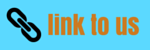# Light Class 8 Science Practice question CBSE

In this page we have Light Class 8 Science Practice question CBSE . Hope you like them and do not forget to like , social shar and comment at the end of the page.

## PRACTICE Question

Question 1.
What do you mean by lateral inversion?
Question 2.
How will you experimentally locate the image of an object made by a plane mirror?

Question 3.
Draw a ray diagram to locate the image of an object placed 5 cm in front of a plane mirror?

Question 4.
Differentiate between regular and diffused reflection. Draw diagram to show the two types of reflection?

Question 5.
State three uses of plane mirrors

Question 6.
What is Kaleidoscope? State the principle on which it is based?

Question 7.
Distinguish between myopia and hypermetropia?

Question 8.
How many images formed by two mirrors kept at an angle of 550?

Question 9.
Explain persistence of vision?

Question 10.
With the help of neat illustrations, describe the principle focus of spherical lenses?

Question 11.
How is rainbow formed?

Question 12.
What is the blind spot? where it is located?

Question 13.
Describe, giving reasons, the path of a ray of light incident on a glass slab takes till it emerges from the slab?

Question 14.
What do you mean by accommodation of the eye? What gives the eye the power of accommodation?

Question 15.
A coin kept at the bottom of a glass bowl full of water appears to be higher than it actually is? With the help of labeled diagram, explain why this happen?